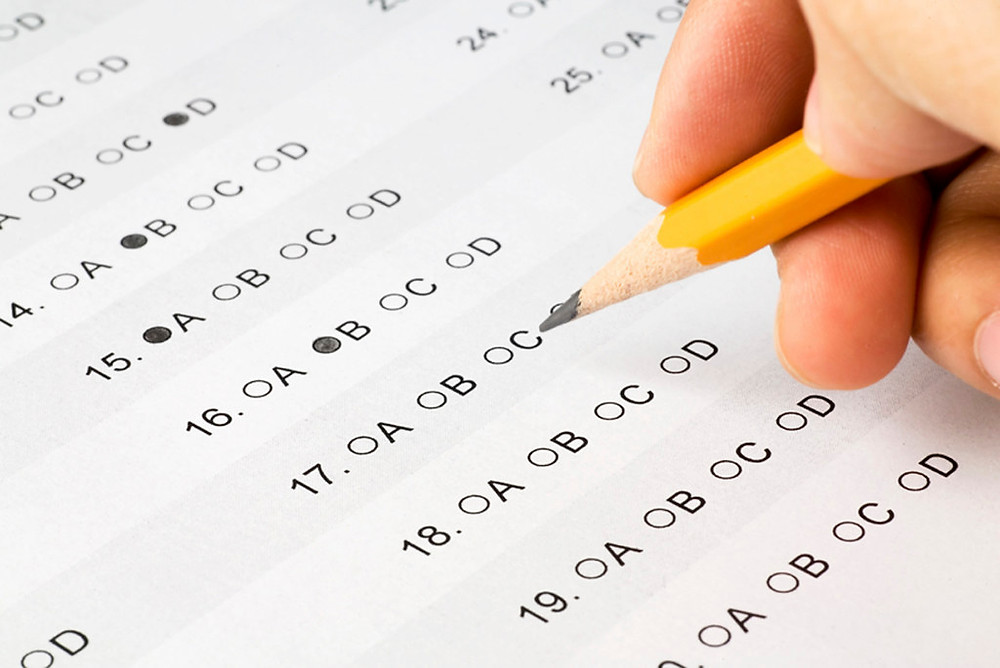# The usefulness of multiple choiceA level papers now have multiple choice sections and GCSE science papers either have multiple choice papers (such as Cambridge International) or a certain proportion of multiple choice questions.

It is usually thought that multiple choice questions are easy. In fact, they can be very difficult. Why? Because the incorrect answers are based on common misconceptions and traps that people fall into if they are not careful.

Take this question for instance...The answer is C. The exam notes state:

"Students struggled with this question (30.2% correct). Many did not appreciate that there are two uncertainties as two readings of the balance are taken to find a change in mass. Many did not realise that the uncertainty is with the mass of the solute itself."

So the question had two uncertainties and if the student pick up on that, then they would have obtained an answer that is incorrect. The incorrect numbers were deliberately added as the examiners knew that they would be the most common wrong answers.

So multiple choice questions actually require you to have a deep understanding of the subject and have a good attention to detail. The exam boards like to do things such as give you values with different units to see if you notice and convert them.

However, remember that not all multiple choice questions are created equal. Some are simple recall questions that you can do in a second. Some are long calculations that may take two - three minutes.

What I find effective is to do multiple choice questions until I hit a slow one and then skip it and move on. That way, I've done as many multiple choice questions as possible in the shortest amount of time and then I know how much time I have for the long and/or difficult ones.

See if this works for you...

#examtechnique